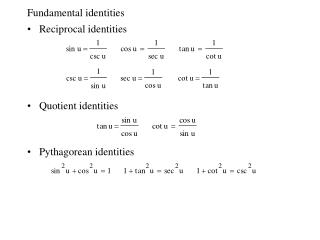Download PresentationFundamental identities

Fundamental identities - PowerPoint PPT Presentation

Fundamental identities. Reciprocal identities Quotient identities Pythagorean identities. Fundamental identities, continued. Cofunction identities Even/Odd identities. Two possible ways to describe a right triangle. Using fundamental identities--an example.I am the owner, or an agent authorized to act on behalf of the owner, of the copyrighted work described.
Download PresentationFundamental identities

Download Policy: Content on the Website is provided to you AS IS for your information and personal use and may not be sold / licensed / shared on other websites without getting consent from its author.While downloading, if for some reason you are not able to download a presentation, the publisher may have deleted the file from their server.

- - - - - - - - - - - - - - - - - - - - - - - - - - E N D - - - - - - - - - - - - - - - - - - - - - - - - - -
Presentation Transcript
1. Fundamental identities • Reciprocal identities • Quotient identities • Pythagorean identities

2. Fundamental identities, continued • Cofunction identities • Even/Odd identities

3. Two possible ways to describe a right triangle

4. Using fundamental identities--an example • Use fundamental identities to verify the trig identity • We simplify the left-side of the identity.

5. Guidelines for verifying trigonometric identities • Work with one side of the equation at a time. • Look to factor an expression, add fractions, square a binomial, or create a monomial denominator. • Look to use the fundamental identities. Note which functions are in the final expression you want. Sines and cosines pair up well, as do secants and tangents, and cosecants and cotangents. • If the preceding guidelines do not help, then try converting all terms to sines and cosines. • Always try something.

6. Practice problems--verify the identity

7. Solving trigonometric equations • If you are given a trigonometric equation, your task is to manipulate it using algebra and the fundamental trigonometric identities until you can write an equation of the form • Example. Solve 2∙cos x = 1. Clearly, this is equivalent to cos x = 1/2. To solve for x, note that there are two solutions in [0, 2), namely /3 and 5/3. Also, because cos x has a period of 2, there are infinitely many other solutions, which can be written as • Since the latter solution gives all possible solutions of the equation, it is called the general solution.

8. Graphical approaches to solving cos x = 1/2 • The graph indicates how an infinite number of solutions can occur. • Also, the unit circle shows infinitely many solutions occur.      

9. Solving trigonometric equations--an example • Solve (tan 3x)(tan x) = tan 3x. This equation can be rewritten as (tan 3x)(tan x – 1) = 0. Next, set the factors to zero. We have • To solve (i) for 3x in the interval [0, ), we have 3x = 0. In general, we have 3x = n so that x = n/3, n an integer. • To solve (ii) for x in the interval [0, ), we have x = /4. In general, we have x = /4 + n, n an integer.

10. Solving trigonometric equations--another example • Solve csc x + cot x = 1. First, let's convert to sines and cosines. We obtain • Since there is no obvious way to solve 1 + cos x = sin x directly, we will try squaring both sides in the hope that the Pythagorean identity will result in a simplification. Of course, we know that squaring may introduce extraneous solutions. We have • After canceling 1, the latter equation becomes • cos x = 0 yields /2 + 2n, n an integer, cos x = –1 yields  + 2n, n an integer, which is extraneous since csc x and cot x are undefined at x = .

11. Solving trigonometric equations--using inverse functions • Solve • The general solution is:

12. Guidelines for solving trigonometric equations • Try to isolate the trigonometric function on one side of the equation. • Look to use standard techniques such as collecting like terms and factoring (or use the quadratic formula). • Look to use the fundamental identities. • To solve equations that contain forms such as sin kx or cos kx, first solve for kx and then divide by k. • If you can't get a solution using exact values, use inverse trigonometric functions to solve.

13. Practice problems--solving trigonometric equations

14. Application of sum and difference formulas • Sum and Difference Formulas

15. Application of sum and difference formulas--two examples • Find the exact value of • Verify that

16. Double-angle and power-reducing formulas • Double-Angle Formulas • Power-Reducing Formulas (double-angle formulas restated)

17. Using double-angle formulas to solve an equation • Solve cos 2x + cos x = 0. First use the double-angle formula for cos 2x. n an integer

18. Using the power-reducing formulas • Rewrite sin4x in terms of first powers of the cosines of multiple angles.

19. Other formulas not covered • Half-Angle formulas, Product-to-Sum Formulas, and Sum-to-Product Formulas are interesting, but will not be covered in this course.

20. Solution of number 7 from previous slide • Let u = x/2, then becomes • Using a double angle formula, we have • This becomes which factors as • We have two cases (i) sin u = −1/2, (ii) sin u = 1. • (i) yields u = 7π/6, u = 11π/6, (ii) yields u = π/2. • Thus x = 7π/3, x = 11π/3, x = π , which are all the solutions in [0, 4π).